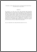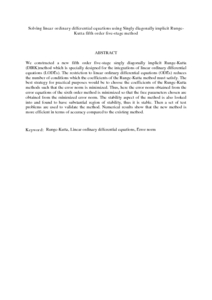# Solving linear ordinary differential equations using Singly diagonally implicit Runge-Kutta fifth order five-stage method

## Citation

Ismail, Fudziah and Che' Jawias, Nur Izzati and Suleiman, Mohamed and Jaafar, Azmi (2009) Solving linear ordinary differential equations using Singly diagonally implicit Runge-Kutta fifth order five-stage method. WSEAS Transactions on Mathematics, 8 (8). pp. 393-402. ISSN 1109-2769

## Abstract

We constructed a new fifth order five-stage singly diagonally implicit Runge-Kutta (DIRK)method which is specially designed for the integrations of linear ordinary differential equations (LODEs). The restriction to linear ordinary differential equations (ODEs) reduces the number of conditions which the coefficients of the Runge-Kutta method must satisfy. The best strategy for practical purposes would be to choose the coefficients of the Runge-Kutta methods such that the error norm is minimized. Thus, here the error norm obtained from the error equations of the sixth order method is minimized so that the free parameters chosen are obtained from the minimized error norm. The stability aspect of the method is also looked into and found to have substantial region of stability, thus it is stable. Then a set of test problems are used to validate the method. Numerical results show that the new method is more efficient in terms of accuracy compared to the existing method.Preview
PDF (Abstract)
Solving linear ordinary differential equations using Singly diagonally implicit Runge.pdfView Item